# Q2) A canal of trapezoidal section has bed width of 8 m and bed slope of...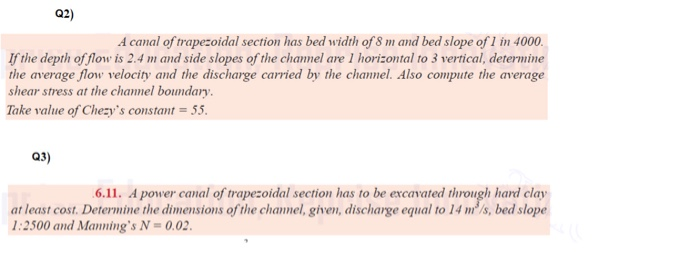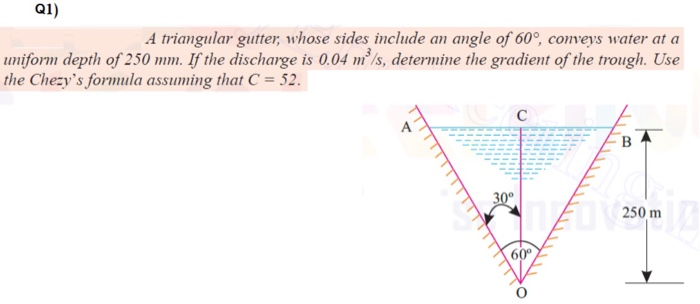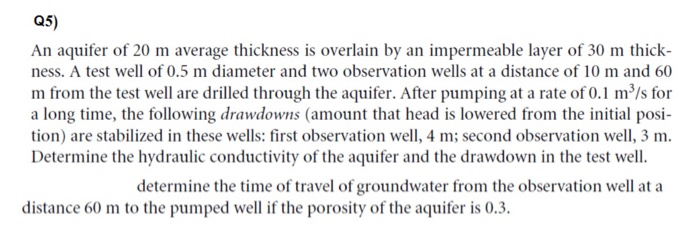Q2) A canal of trapezoidal section has bed width of 8 m and bed slope of 1 in 4000. If the depth of flow is 2.4 m and side slopes of the chamel are 1 horizontal to 3 vertical, determine the average flow velocity and the discharge carried by the channel. Also compute the average shear stress at the channel boundary. Take value of Chezy's constant = 55. Q3) 6.11. A power canal of trapezoidal section has to be excavated through hard clay at least cost. Determine the dimensions of the channel, given, discharge equal to 14 m/s, bed slope 1:2500 and Manning's N = 0.02.
Q1) A triangular gutter, whose sides include an angle of 60°, conveys water at a uniform depth of 250 mm. If the discharge is 0.04 m/s, determine the gradient of the trough. Use the Chezy's formula assuming that C = 52. с B 30° 250 m 60°
Q5) An aquifer of 20 m average thickness is overlain by an impermeable layer of 30 m thick- ness. A test well of 0.5 m diameter and two observation wells at a distance of 10 m and 60 m from the test well are drilled through the aquifer. After pumping at a rate of 0.1 m²/s for a long time, the following drawdowns (amount that head is lowered from the initial posi- tion) are stabilized in these wells: first observation well, 4 m; second observation well, 3 m. Determine the hydraulic conductivity of the aquifer and the drawdown in the test well. determine the time of travel of groundwater from the observation well at a distance 60 m to the pumped well if the porosity of the aquifer is 0.3.

Complete solution for question 1 is given below.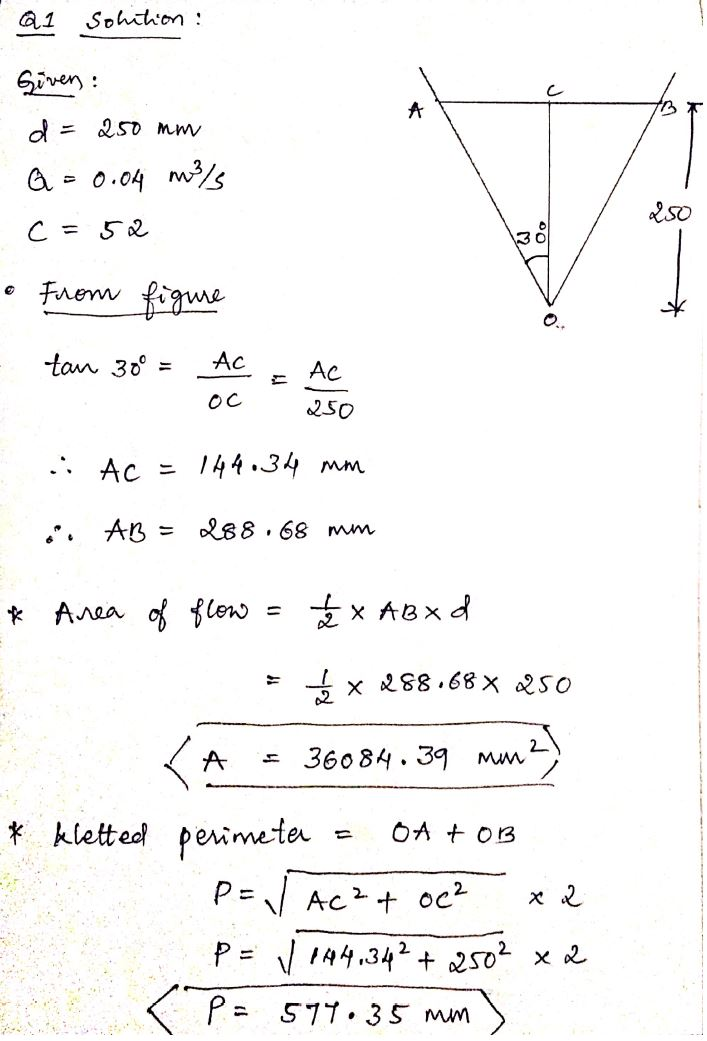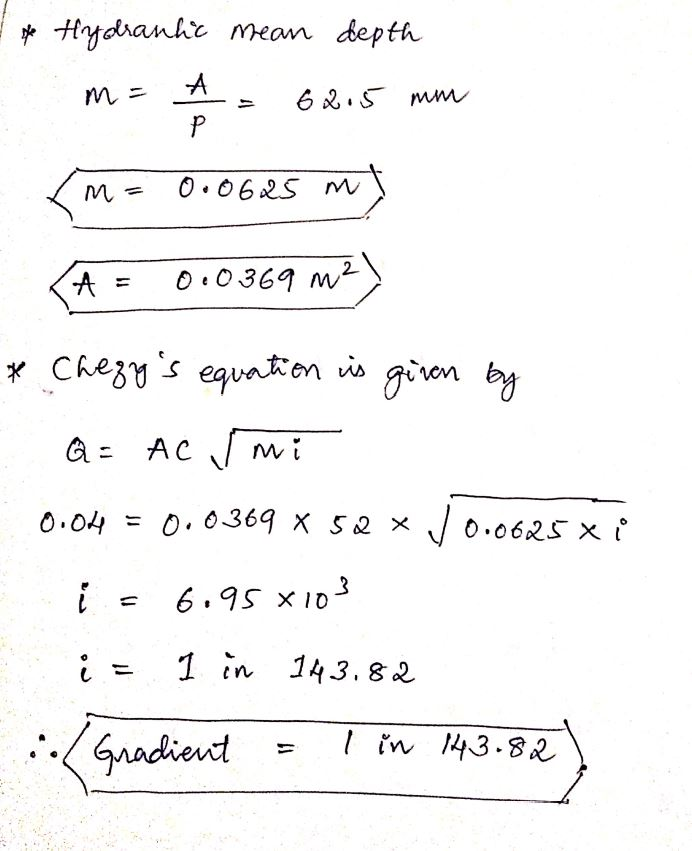#### Earn Coin

Coins can be redeemed for fabulous gifts.

Similar Homework Help Questions
• ### 8. A rectangular channel section has a change in slope as shown in figure below. The chamel is 4m...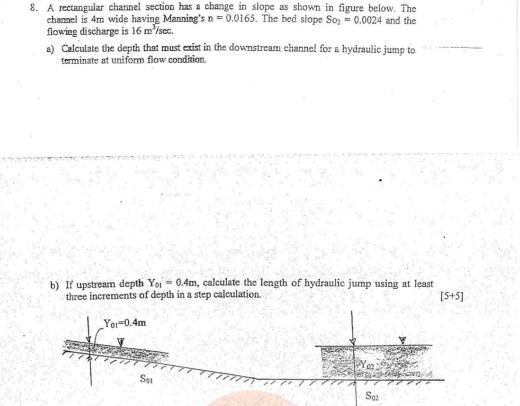please solve this problem 8. A rectangular channel section has a change in slope as shown in figure below. The chamel is 4m wide having Manning's n = 0.0165. The bed siope So,-0.0024 and the flowing discharge is 16 m,sec. a) Calculate the depth that must exrist in the downstream channe!l for a hydraulic jump to terminate at uniform fiow condition. b) If upstream depth Yoi 0.4m, calculate the length of hydraucump using at least three increments of depth in...

• ### A 2.44 wide channel with a bed slope of 0.0004 has a depth of flow of...

A 2.44 wide channel with a bed slope of 0.0004 has a depth of flow of 0.61 m. (a) Determine the discharge of the channel. The manning coefficient is 0.015. (b) Determine the normal depth if the channel has a flow rate of 2.85 m3/s. (c) Determine the cross section of greatest hydraulic efficiency for a trapezoidal channel if the design discharge is 10 m3/s, the channel slope is 0.0052 and manning’s n = 0.025.

• ### Question 6 A concrete-lined trapezoidal channel with uniform flow has a normal depth of 1.5 m. The base width is 4 m and the side slopes are equal at 1 vertical to 2 horizontal. Mannin‘s n-0015....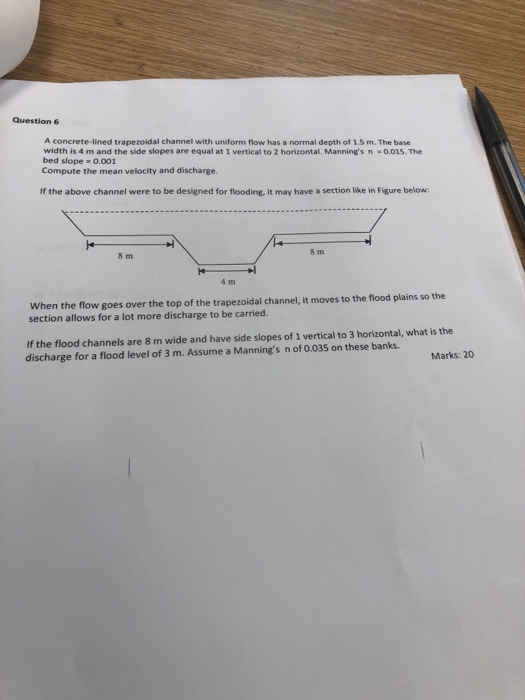Question 6 A concrete-lined trapezoidal channel with uniform flow has a normal depth of 1.5 m. The base width is 4 m and the side slopes are equal at 1 vertical to 2 horizontal. Mannin‘s n-0015. The bed slope 0.001 Compute the mean velocity and discharge. If the above channel were to be designed for flooding, it may have a section like in Figure below 8 m 8 m 4 m When the flow goes over the top of the...

• ### Water flows down a rectangular channel that has a width of 2.2 m, a Manning's n...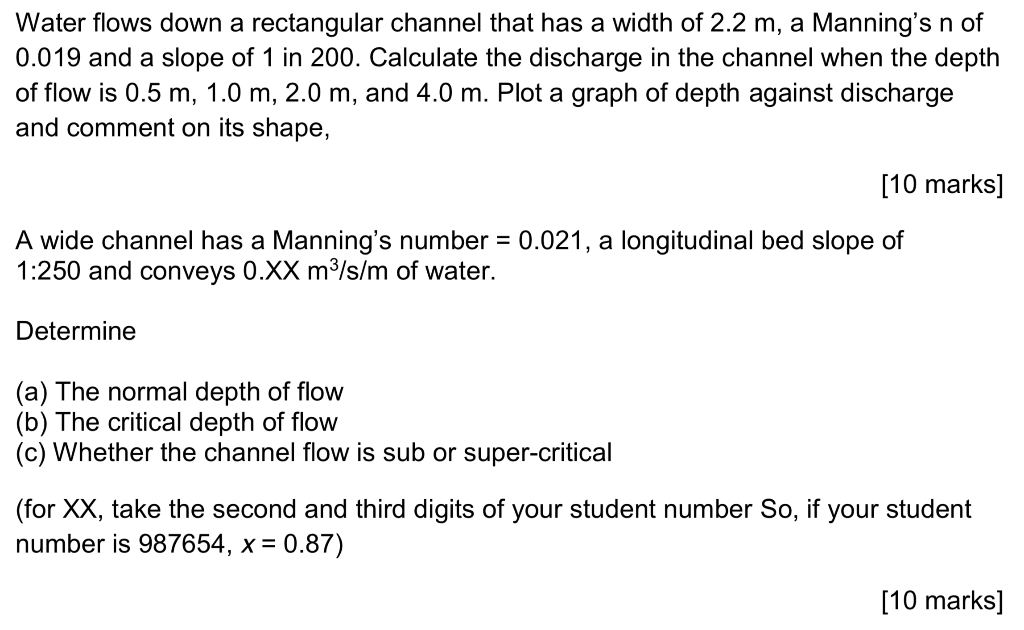Water flows down a rectangular channel that has a width of 2.2 m, a Manning's n of 0.019 and a slope of 1 in 200. Calculate the discharge in the channel when the depth of flow is 0.5 m, 1.0 m, 2.0 m, and 4.0 m. Plot a graph of depth against discharge and comment on its shape, [10 marks] A wide channel has a Manning's number = 0.021, a longitudinal bed slope of 1:250 and conveys 0.XX m3/s/m of...

• ### A trapezoidal channel has a bottom width, b, of 10.0 m and a side slope ratio...

A trapezoidal channel has a bottom width, b, of 10.0 m and a side slope ratio of 3:1. The Manning’s n of the channel is 0.025, and it is laid on a slope of 0.001. If the water depth is 2 m at the downstream end, write and submit an R script to compute the water surface profile for a discharge of 30 m3 /s. Also submit a plot of the water profile in elevation (m) for 1000 m channel...

• ### A trapezoidal channel has a bottom width 10 ft and side slope of 2H:1V. It carries...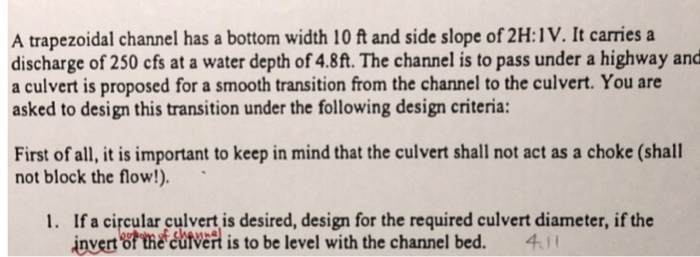A trapezoidal channel has a bottom width 10 ft and side slope of 2H:1V. It carries a discharge of 250 cfs at a water depth of 4.8ft. The channel is to pass under a highway and a culvert is proposed for a smooth transition from the channel to the culvert. You are asked to design this transition under the following design criteria: First of all, it is important to keep in mind that the culvert shall not act as a...

• ### #4 [5 points] A trapezoidal channel of bottom width 25 ft and side slope 1:2.5 carries...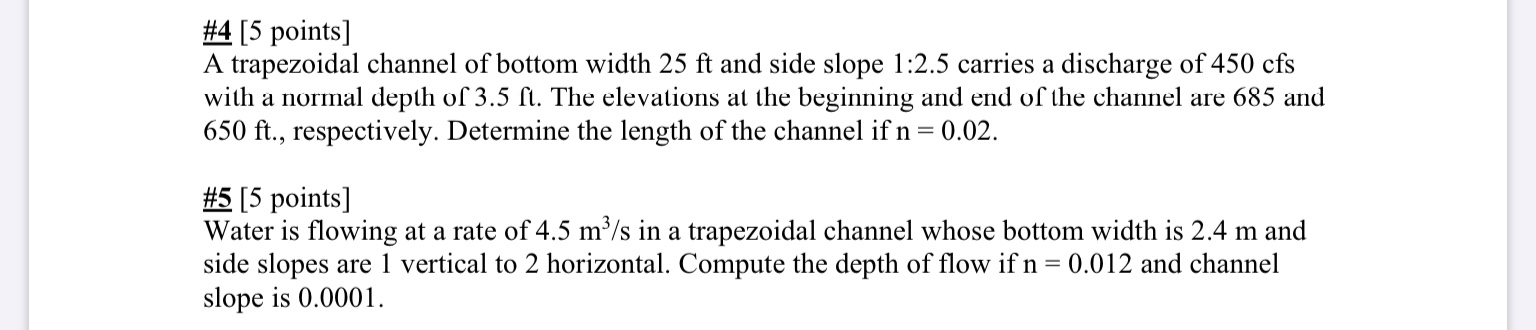#4 [5 points] A trapezoidal channel of bottom width 25 ft and side slope 1:2.5 carries a discharge of 450 cfs with a normal depth of 3.5 ft. The elevations at the beginning and end of the channel are 685 and 650 ft., respectively. Determine the length of the channel if n = 0.02. #5 [5 points] Water is flowing at a rate of 4.5 m3/s in a trapezoidal channel whose bottom width is 2.4 m and side slopes are...

• ### (5) Water flows along a trapezoidal channel with base width B=1.5 m, side slope 1:1 (horizontal...

(5) Water flows along a trapezoidal channel with base width B=1.5 m, side slope 1:1 (horizontal to vertical) and depth D=0.7 m. It is controlled downstream by a sharp crested weir that has a crest height of H=2.4 m above the channel bed and a crest width equal to that of the base width. Calculate the flow rate in the channel (check any assumptions you make).

• ### Problem # 2 A discharge of 4800 m/s occurs in an approximately rectangular channel with a depth of 10 m and a width of 50 m. The channel slopes at a rate of 1/2000. The bed consists of fine sand (ds-...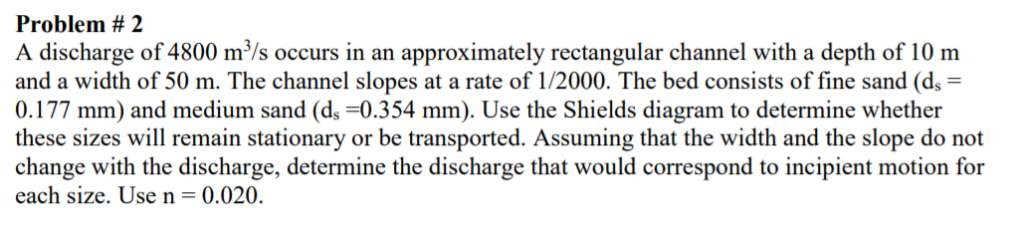Problem # 2 A discharge of 4800 m/s occurs in an approximately rectangular channel with a depth of 10 m and a width of 50 m. The channel slopes at a rate of 1/2000. The bed consists of fine sand (ds- 0.177 mm) and medium sand (ds-0.354 mm). Use the Shields diagram to determine whether these sizes will remain stationary or be transported. Assuming that the width and the slope do not change with the discharge, determine the discharge that...

• ### Q1. For a uniform flow, a trapezoidal channel has a base width b = 8 m...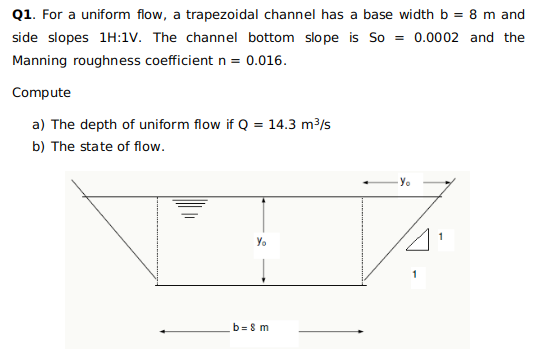Q1. For a uniform flow, a trapezoidal channel has a base width b = 8 m and side slopes 11:1V. The channel bottom slope is so = 0.0002 and the Manning roughness coefficient n = 0.016. Compute a) The depth of uniform flow if Q = 14.3 m3/s b) The state of flow. yo У. b= 8 m